Copyright © Michael Richmond. This work is licensed under a Creative Commons License.

# Precession, Proper Motion, and Angular Separation

It's bad enough that there are several different coordinate systems used by astronomers. But what's even worse is that, even when you have chosen one coordinate system, the coordinates of stars in that system will change over time. Fortunately, these changes are usually very slow, acting over decades or centuries, but you must be aware of them to compare accurately measurements made in the nineteen-fifties to measurements made today.

After discussing the two major reasons for changes in stellar positions, I'll show you how to calculate the angular distance between two locations on the sky. Most planetarium programs will do this for you, but it never hurts to know what's going on inside the box.

#### Precession

Let's watch the stars as they glide through the sky over the course of a night:

The constellations appear to circle around the Pole Star, Polaris, at the end of the Little Dipper's handle.

```

Q:  Why do the stars appear to rotate around this point?

Q:  What is the (RA, Dec) position of the North Celestial Pole?

```

Over the course of any single human lifetime, that's the way it goes. But what would happen if we could watch the sky night after night for centuries? For example, let's jump forward in time one thousand years, to March 12, 3005, and once again watch the Rochester skies after sunset.

```

Q:  How do the stars and constellations move now?

```

Let's jump forward even farther, to the year 7005, a round five millenia into the future.

```

Q:  What happens now?

Q:  What is going on???

```

The reason for the change in the apparent motion of the stars has nothing to do with the stars themselves; instead, it's all due to the Earth. As the Earth spins around its axis, it feels gentle gravitational tugs (torques) due to the Moon and to the Sun. In very simple terms, the torques are caused by the differential gravitational force exerted on the near and far sides of the Earth's equatorial bulge: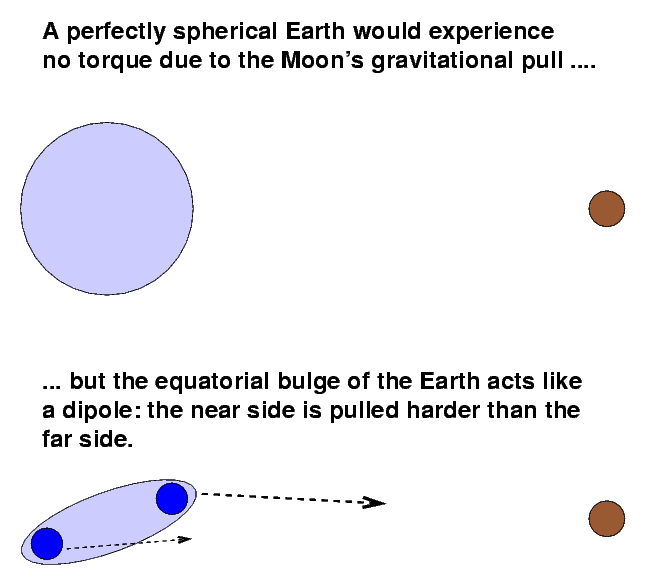The torque causes the spinning Earth to precess: its axis of rotation slowly rotates. It takes about 26,000 years to make one complete cycle.

This illustration from H. A. Rey's book The Stars: A New Way to See Them illustrates the change in the "pole star" over the period of the precession of the equinoxes.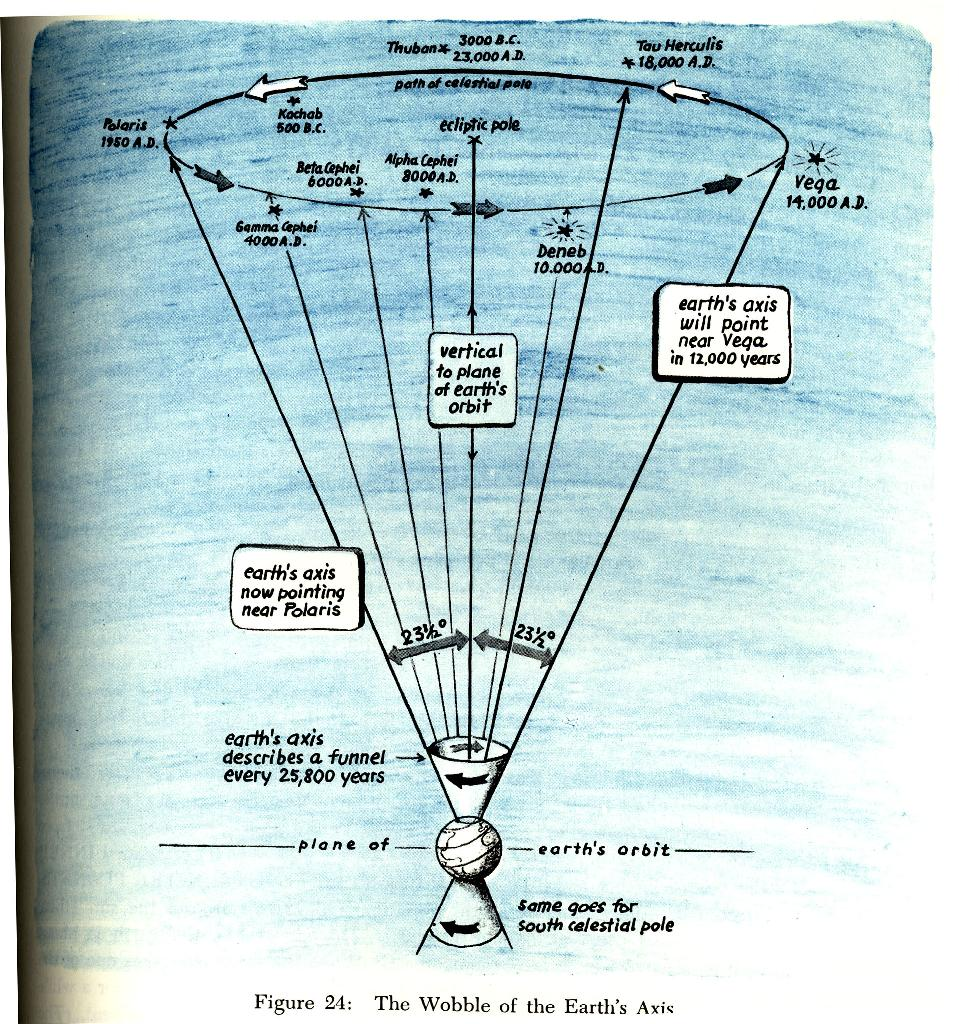The Stars: A New Way to See Them by H. A. Rey, published by Houghton Mifflin (1976) -- it's my FAVORITE constellation book!

Astronomers want their (RA, Dec) coordinate system to be aligned with the Earth's axis of rotation ... so as the Earth precesses, astronomers move their coordinate system to follow it. That means that the (RA, Dec) coordinates will slowly drift across the background of stars. Look at the Pleiades over the period 1950 to 2000:

It's a big pain to re-publish the coordinates of all the stars and galaxies, so astronomers only do it occasionally. There was one big set of catalogs produced for the direction of the Earth's axis in 1950; those coordinates are referred to as (1950) or (B1950) or "equinox 1950".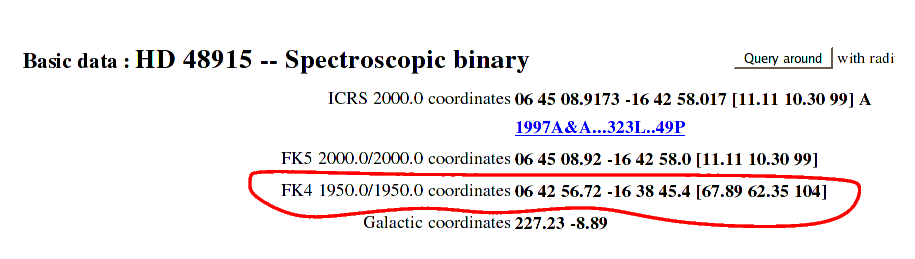Another big set of catalogs was created fifty years later, using the direction of the Earth's axis in the year 2000. These are denoted by (2000) or (J2000) or "equinox 2000".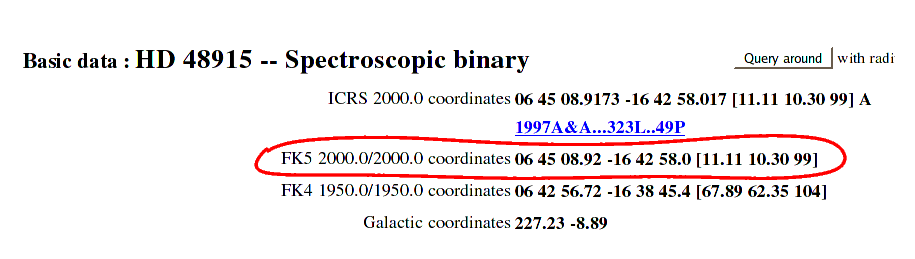For our purposes, the "equinox 2000" positions are preferred. Please use them unless directed otherwise. You can find tools to convert coordinates from one equinox to another in many places -- most planetarium programs will do it, for example. You may have to prepend the letter "B" to an equinox when you type them into a converter -- so that you refer to a star with coordinates of equinox 1923 as having equinox B1923.

#### Proper Motion

Suppose that we just take a picture of the stars, like this one of the Big Dipper: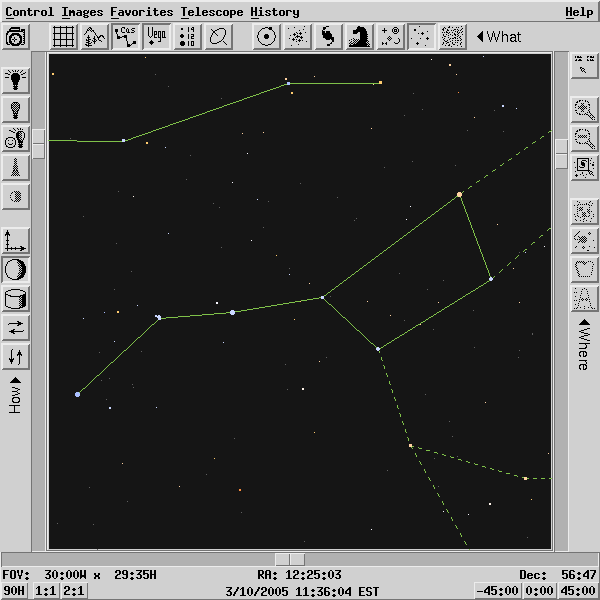If all we care about is the relative positions of the stars -- which create the shapes of the constellations -- then we can ignore precession; precession only changes the coordinates assigned to the stars, not their relative positions.

So, we might expect the Big Dipper to remain unchanged in the sky as the years go by ... but is it really? Let's step into the Wayforward Machine and set the dial for steps of 1000 years:

Hey! The stars are all wandering away from their original positions! The effect is even more obvious if you look at a movie of Ursa Major from 100,000 BC to 100,000 AD ; thanks very much to Richard Pogge at Ohio State University for making this available.

You might also run your own simulations with Tony Dunn's proper motion simulator (runs in a browser).

```
Q:  Why are the stars moving individually in
different directions?

```

Astronomers call this very gradual glide across the sky the proper motion of a star. It depends on two factors:

• the speed of the star through space relative to the Sun
• the distance of the star

Over the past few hundred years, astronomers have carefully measured the proper motions of thousands of stars. The values are often expressed in units of "arcseconds per year," or sometimes "milliarcseconds per year." In the example below, there are two values given:

• the proper motion in Right Ascension, in milliarcseconds per year
• the proper motion in Declination, in milliarcseconds per year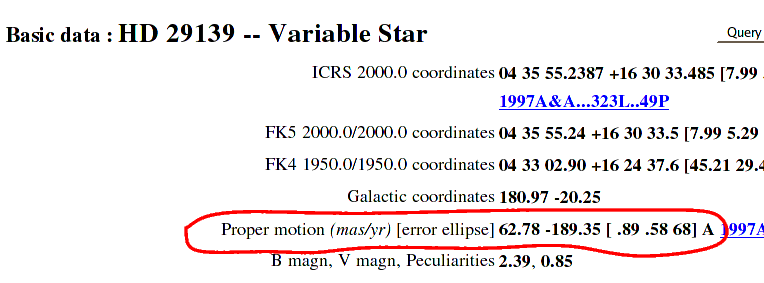```
Q:  How far in RA  should Aldebaran move in 50 years?

How far in Dec should Aldebaran move in 50 years?

```

Hmmmm. Does this mean we can just add 3.1 arcseconds to the RA value for the star in example above? How would that work? That brings up the whole topic of the arithmetic of angular distances.

#### Calculating the angular separation between objects

Doing geometry on a plane is easy: you learned the rules in high school.

• the area of a circle is π R2
• the sum of the angles of a triangle is 180 degrees
etc.

If you want to know the distance between two points ...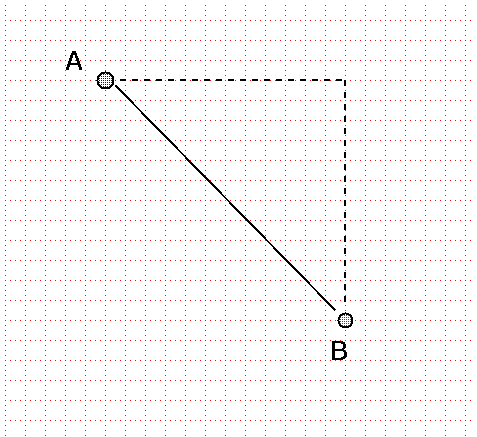```
Q:  How do you find the distance between points
A and B in the figure above?

```

Astronomers, however, don't deal with objects sitting on a plane. From our point of view on Earth, stars and planets appear to be projected onto a SPHERE surrounding the Earth. The coordinate systems we use, such as the equatorial (RA and Dec) or galactic (galactic latitude and longitude), all involve positions on this celestial sphere.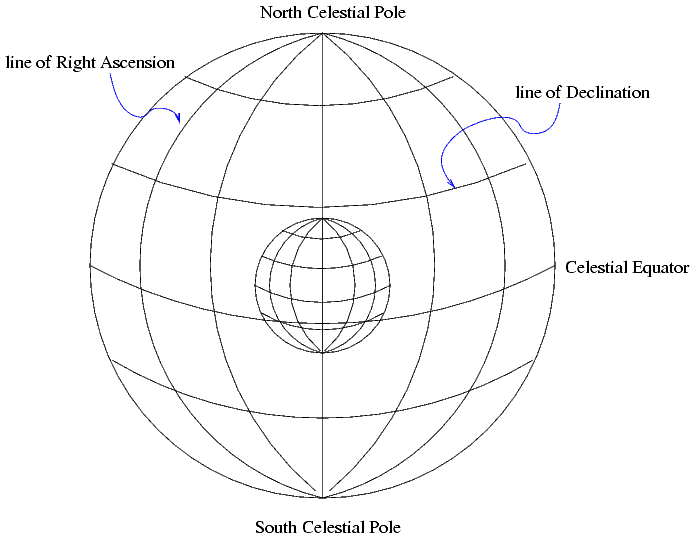Geometry on a sphere does NOT follow the same rules as geometry on a plane. For example,

• the area of a circle is MORE than π R2
• the sum of the angles in a triangle is MORE than 180 degrees
etc.

In order to determine the distances between objects on the celestial sphere, we need to use spherical trigonometry. There are entire books devoted to this rather arcane subject, such as

• Textbook on Spherical Astronomy by W. M. Smart
• Computational Spherical Astronomy by L. G. Taff
• Spherical Astronomy by R. M. Green

I will provide here just a few of the most basic rules.

First, note that angles which run purely North-South are easy to understand: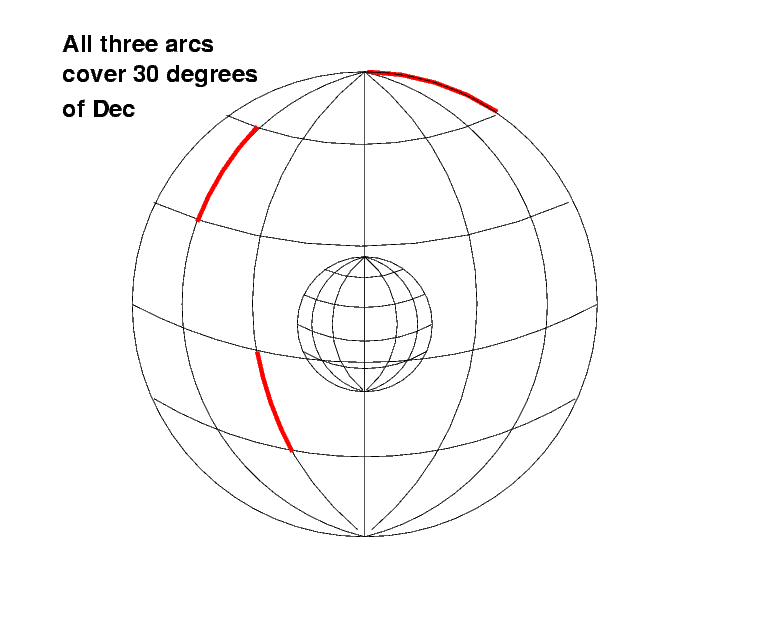```
Q:  Are all three red arcs the same length?

```

So calculating arclengths between two positions which differ only in Dec is easy. For example,

```

A   has    Dec = +30:23:34   =  30.3928  degrees
B          Dec = +30:18:12   =  30.3033  degrees

delta Dec  =  (30.3928 deg  -  30.3033 deg)

=  0.0894 deg

=  00:05:22

=  0 degrees, 5 arcminutes, 22 arcseconds

```

But angles which run parallel to the equator of the coordinate system are more complicated....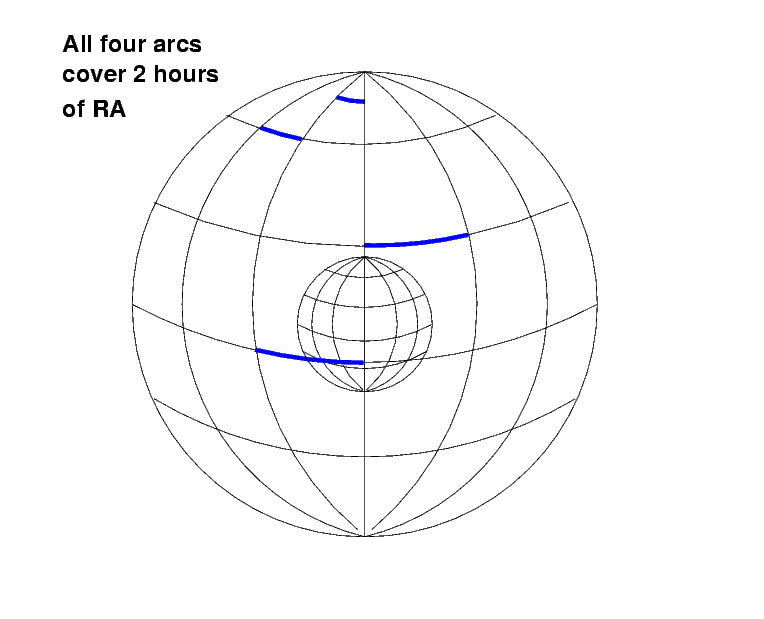```
Q:  Are all four blue arcs the same length?

```

The arclength of a circle which is parallel to the celestial equator changes with Declination:

• the arclength is longest around the celestial equator
• it shinks as one approaches the poles
• and reaches zero right at the poles

One can calculate the arclength of an angle between two points which have the same Dec by correcting for this factor: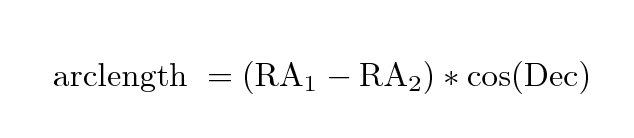For example, if two stars have the same Declination, and differ only in RA,

```

A  has   RA = 02:33:48.7       and  Dec = +20:00:00
B        RA = 02:02:31.3       and  Dec = +20:00:00

First, convert the RA to decimal degrees in two steps,
for both stars.

A    RA = 02 hours    33 minutes        48.7 seconds
=  2 hours + (33/60) hours  + (48.7/3600) hours
=  2.5635 hours

There are 24 hours of RA around the sky, which equal
360 degrees of RA.  So each hour of RA must equal 15 degrees.

A    RA = (2.5635 hours) * (15 degrees/hour of RA)
=  38.4529  degrees

B    RA = 02 hours    02 minutes        31.3 seconds
=  2.0420 hours
=  30.6304  degrees

Now, calculate the difference in RA, including the correction
factor based on the Declination.

delta RA   =  (38.4529 deg  -  30.6403 deg) * cos(20.0000)

=  (7.8122 deg) * (0.9397)

=   7.3411 deg

```

If two points are very close together on the sky -- within a degree or less -- than one can calculate approximately the distance between them by adding this little correction factor to the Pythagorean formula. Make sure that both RA and Dec are expressed in decimal degrees, and then compute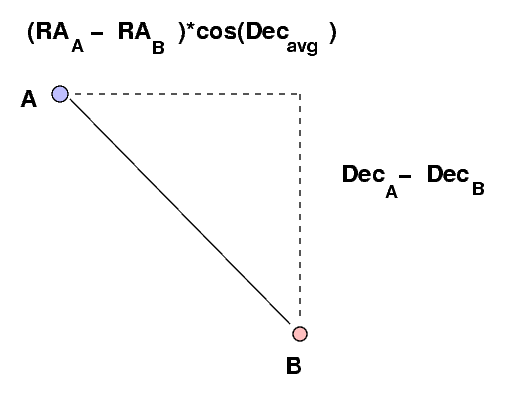But for the general case of two locations separated by an arbitrary distance, one must fall back upon the full formula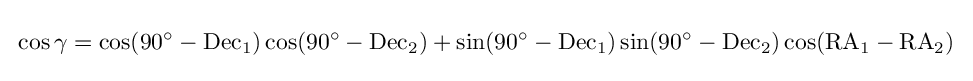to calculate the arclength γ between the two locations.

#### Homework

1. The Bonner Durchmusterung (or BD for short) was the first really big star catalog. It was compiled in just a few years by a team of four people, led by Friedrich Argelander at the Bonn Observatory. As you can see from the title page of the fourth volume above, it was created in the mid-1800s. The positions are given for the equinox 1855. This tiny extract from one page shows the format of the catalog: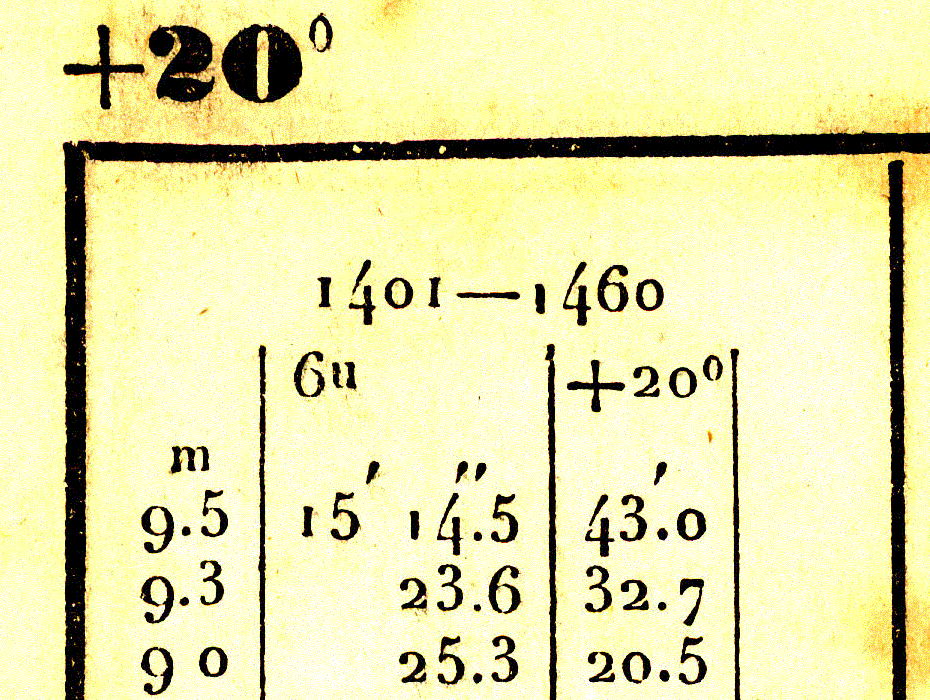The top of the page shows that stars on this page belong to zone +20; that means they are all in a strip around the sky near Declination = +20 degrees. Below that is one column from the page: it shows the list of stars numbered 1401 to 1460 in the zone +20: the first star is called BD +20 1401, the second star BD +20 1402, and so forth. The leftmost number is the magnitude of the star -- small numbers mean bright stars, big numbers mean faint stars (we'll discuss magnitudes later). The middle numbers give the Right Ascension of the star. All the stars on this page fall into RA = 6 hours; the numbers below show the minutes and seconds of RA. The right-most numbers provide the declination of the star: all have Dec = +20, but the minutes and fractions of a minute are listed for each star.

Why does the catalog have this somewhat confusing, very abbreviated format? Think about it for a moment. The entire catalog runs to eight (I think) volumes each over three hundred pages long!

Thus, the second star in the list has

• name BD +20 1402
• magnitude 9.3
• Right Ascension 6 hours 15 minutes 23.6 seconds = 06:15:23.6
• Declination +20 degrees 32.7 minutes = +20:32:42

Look at the stars on this page of the catalog (click on the image for a larger version):

1. Find the star BD +20 1409
2. What is its magnitude?
3. Write down the RA and Dec of this star from the catalog. Express each one in HH:MM:SS format.
4. Recall that the BD provides positions in equinox 1855. Convert the coordinates to the equinox 2000, so that we can compare the position to current star charts and maps.

2. Use SIMBAD's query tool to look up more information about this particular star. You should be able to search by its name ("BD+20 1409") or by its position (you would type something like "01 23 03.4 +20 12 08").
1. This star has another name, based on a catalog with abbreviation "HD" (it stands for "Henry Draper", who paid for the catalog). What is the star's HD number?
2. What is the star's actual position listed by SIMBAD for equinox 2000? Pick the "ICRS 2000.0" coordinates.

(This is the hard part -- just do your best)

1. What is the angular distance between your predicted position of this star in equinox 2000 (based on the precessed value of the BD position) and the actual position listed by SIMBAD? Express your answer in arcseconds of Right Ascension, and arcseconds of Declination.
2. The SIMBAD listing shows the proper motion of this star, in units of milliarcseconds per year. How many arcseconds should it have moved through the sky between the year 1855 and the year 2000?
3. Does the proper motion of the star explain the difference between your predicted position and the actual position? (Don't worry too much if it doesn't; the BD positions had a small amount of random error, which might also explain small differences)Copyright © Michael Richmond. This work is licensed under a Creative Commons License.